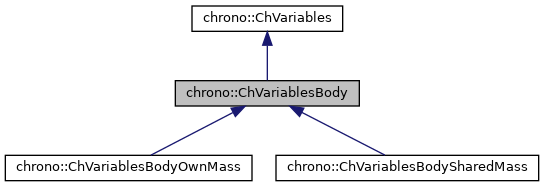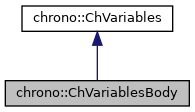chrono::ChVariablesBody Class Referenceabstract

## Description

Specialized class for representing a 6-DOF item for a system, that is a 3D rigid body, with mass matrix and associate variables (a 6 element vector, ex.speed) This is an abstract class, specialized for example in ChVariablesBodyOwnMass and ChVariablesBodySharedMass.

#include <ChVariablesBody.h>

Inheritance diagram for chrono::ChVariablesBody:[legend]
Collaboration diagram for chrono::ChVariablesBody:[legend]

## Public Member Functions

ChVariablesBodyoperator= (const ChVariablesBody &other)
Assignment operator: copy from other object.

virtual double GetBodyMass () const =0
Get the mass associated with translation of body.

virtual ChMatrix33GetBodyInertia ()=0
Access the 3x3 inertia matrix.

virtual const ChMatrix33GetBodyInertia () const =0

virtual ChMatrix33GetBodyInvInertia ()=0
Access the 3x3 inertia matrix inverted.

virtual const ChMatrix33GetBodyInvInertia () const =0

virtual int Get_ndof () const override
The number of scalar variables in the vector qb (dof=degrees of freedom)

void * GetUserData ()

void SetUserData (void *mdata)Public Member Functions inherited from chrono::ChVariables
ChVariables (int m_ndof)

ChVariablesoperator= (const ChVariables &other)
Assignment operator: copy from other object.

void SetDisabled (bool mdis)
Deactivates/freezes the variable (these variables won't be modified by the system solver).

bool IsDisabled () const
Check if the variables have been deactivated (these variables won't be modified by the system solver).

bool IsActive () const
Check if these variables are currently active. More...

ChVectorRef Get_qb ()
Returns reference to qb, body-relative part of degrees of freedom q in system: More...

virtual void Compute_fb ()
Compute fb, body-relative part of known vector f in system. More...

ChVectorRef Get_fb ()
Returns reference to fb, body-relative part of known vector f in system. More...

virtual void Compute_invMb_v (ChVectorRef result, ChVectorConstRef vect) const =0
Computes the product of the inverse mass matrix by a vector, and store in result: result = [invMb]*vect.

virtual void Compute_inc_invMb_v (ChVectorRef result, ChVectorConstRef vect) const =0
Computes the product of the inverse mass matrix by a vector, and increment result: result += [invMb]*vect.

virtual void Compute_inc_Mb_v (ChVectorRef result, ChVectorConstRef vect) const =0
Computes the product of the mass matrix by a vector, and increment result: result = [Mb]*vect.

virtual void MultiplyAndAdd (ChVectorRef result, ChVectorConstRef vect, const double c_a) const =0
Computes the product of the corresponding block in the system matrix (ie. More...

virtual void DiagonalAdd (ChVectorRef result, const double c_a) const =0
Add the diagonal of the mass matrix scaled by c_a, to 'result', as a vector. More...

virtual void Build_M (ChSparseMatrix &storage, int insrow, int inscol, const double c_a)=0
Build the mass submatrix (for these variables) multiplied by c_a, storing it in 'storage' sparse matrix, at given column/row offset. More...

void SetOffset (int moff)
Set offset in global q vector (set automatically by ChSystemDescriptor)

int GetOffset () const
Get offset in global q vector.

virtual void ArchiveOUT (ChArchiveOut &marchive)

virtual void ArchiveIN (ChArchiveIn &marchive)Protected Attributes inherited from chrono::ChVariables